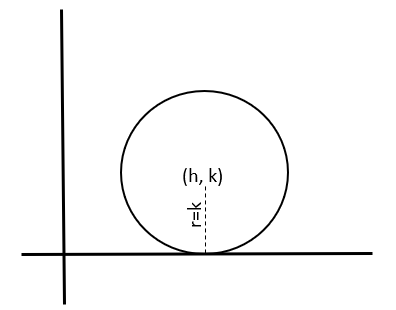Circle1. When the center of circle is origin.→x2+y2 = r2

2. When the center of circle is (h, k).→(x-h)2+(y-k)= r2 ..................(i)

3. When the circle touches x-axis.If the circle touches x-axis then, r=k and putting the value in equation (i)
→(x-h)2+(y-k)= k2

4. When the circle touches y-axis.If the circle touches y-axis then, r=h and putting the value in equation (i)
→(x-h)2+(y-k)= h2

5.When the circle touches both axes.If the circle touches both axes then, r=h=k and putting the value in equation (i)
→(x-h)2+(y-h)= h2
OR,
→(x-k)2+(y-k)= k2
OR,
→(x-r)2+(y-r)= r2

6. When the circle passes through origin.If the circle passes through origin then, r2=h2+k2 and putting the value in equation (i)
(x-h)2+(y-k)2﻿= h2+k2